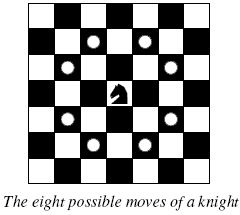# POJ 2488-A Knight's Journey

## A Knight’s Journey

Time Limit
: 1000MS   Memory Limit: 65536K
Total Submissions:36995   Accepted: 12551

Background
The knight is getting bored of seeing the same black and white squares again and again and has decided to make a journey
around the world. Whenever a knight moves, it is two squares in one direction and one square perpendicular to this. The world of a knight is the chessboard he is living on. Our knight lives on a chessboard that has a smaller area than a regular 8 * 8 board, but it is still rectangular. Can you help this adventurous knight to make travel plans?Problem

Find a path such that the knight visits every square once. The knight can start and end on any square of the board.

Input

The input begins with a positive integer n in the first line. The following lines contain n test cases. Each test case consists of a single line with two positive integers p and q, such that 1 <= p * q <= 26. This represents a p * q chessboard, where p describes how many different square numbers 1, … , p exist, q describes how many different square letters exist. These are the first q letters of the Latin alphabet: A, …

Output

The output for every scenario begins with a line containing “Scenario #i:”, where i is the number of the scenario starting at 1. Then print a single line containing the lexicographically first path that visits all squares of the chessboard with knight moves followed by an empty line. The path should be given on a single line by concatenating the names of the visited squares. Each square name consists of a capital letter followed by a number.
If no such path exist, you should output impossible on a single line.
Sample Input

```3
1 1
2 3
4 3`
```

Sample Output

```Scenario #1:
A1

Scenario #2:
impossible

Scenario #3:
A1B3C1A2B4C2A3B1C3A4B2C4
```

(1)以马走日的形式，走过的格子是当前格子和最后到达的格子，中间路过的格子不计在内。楼主也是够了，刚开始的时候居然把中间的格子算上了，结果半天不知道中间格子怎么标记，也是够了。
(2)输入的先是列数后是行数，不注意的话，真的都不知道哪出问题了。
(3)以字典序去搜周围符合条件的点，这个字典序我还真不知道怎么弄，看了各路大神的程序，总算明白了。

`````````
#include<iostream>
#include<string.h>
#include<cstdio>
using namespace std;

const int SIZE = 26;
const int  X = { -2, -2, -1, -1, 1, 1, 2, 2 };
const int Y = { -1, 1, -2, 2, -2, 2, -1, 1 };//字典序的行列值
int StepX[SIZE], StepY[SIZE];//记录走的路径
int Chess[SIZE][SIZE];  //棋盘
int total, row, column, step, Sign;

void DFS(int r, int c)
{
int x, y;
if (Sign == 1)//跟大牛学的终止条件的写法
{
return;
}
step++;
StepX[step] = r;
StepY[step] = c;
if (step == row*column)//终止条件
{
Sign = 1;
return;
}
Chess[r][c] = 1;//走过标记
for (int i = 0; i < 8; i++)
{
y = c + Y[i];
x = r + X[i];//按字典序去搜
if (x <= row && y <= column&&x>0 && y > 0 && Chess[x][y] == 0)
{
DFS(x, y);//递归
step--;
}
}
Chess[r][c] = 0;//当前行列找不到合适的走法回溯
}
int main()
{
int k, t = 1;
cin >> k;
while (k--)
{
step = 0;
Sign = 0;
memset(Chess, 0, sizeof(Chess));
memset(StepX, 0, sizeof(StepX));
memset(StepY, 0, sizeof(StepY));
cin >> column >> row;
cout << "Scenario #" << t++ << ":" << endl;
DFS(1, 1);
if (Sign)
{
for (int i = 1; i < row*column + 1; i++)
cout << char(StepX[i] - 1 + 'A') << StepY[i];
cout << endl;
}
else cout << "impossible" << endl;
if (k != 0)
{
cout << endl;
}

}
return 0;
}
``````